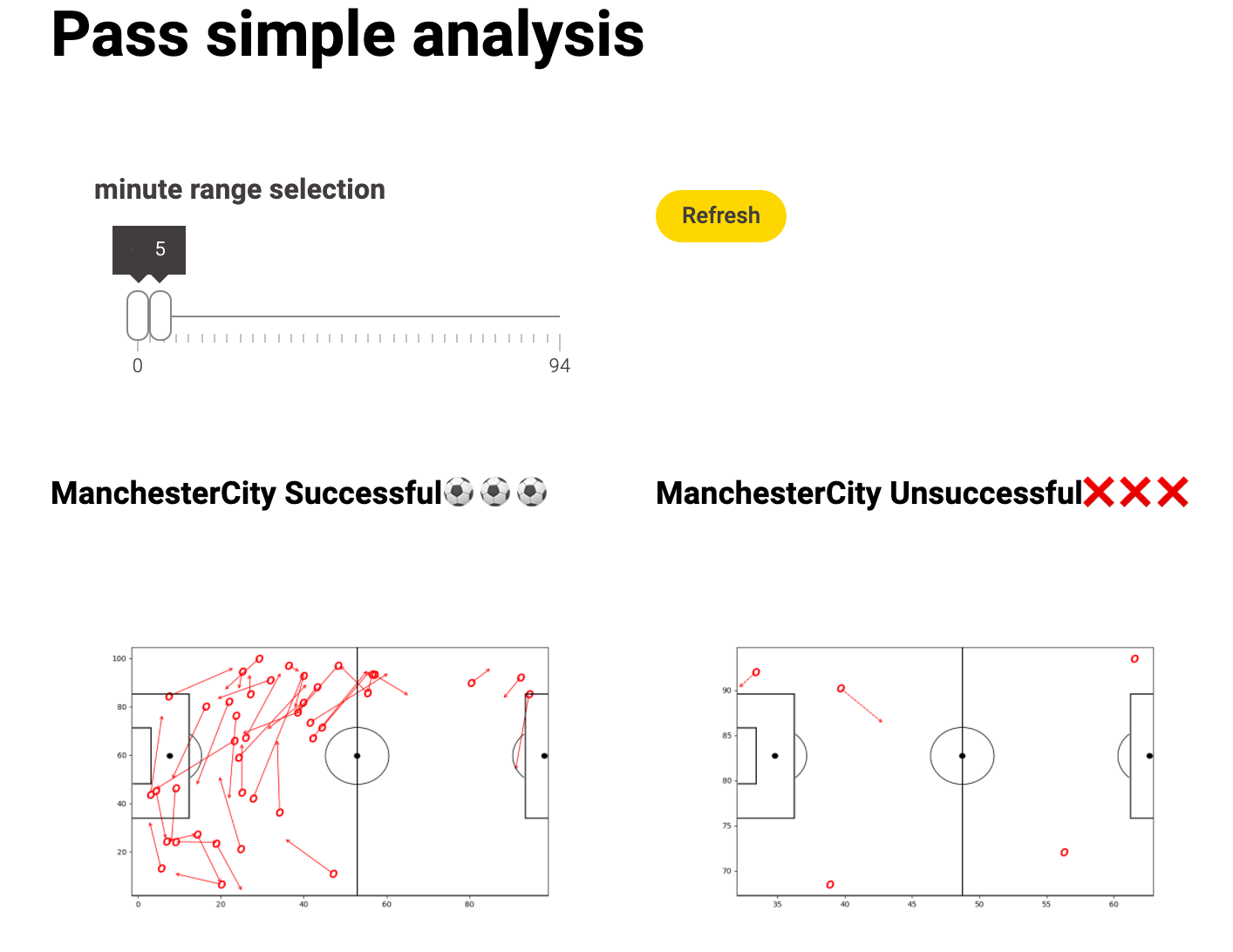# 🥷 [S2] Challenge 07

· 阅读需 2 分钟

Just KNIME It, Season 2 / Challenge 07 reference

## Points worth mentioning​

• I chose a slider to control the time frame.
• Well, in addition to the visualisation of Manchester City's successful passes, I also showed the failed passes, as well as Manchester United's passes. This is because I believe that the success or failure of one team's pass is closely related to that of the other. Of course, only the successful and unsuccessful passes are shown here.### Matplotlib vis​

import knime.scripting.io as knioimport numpy as npimport matplotlib.pyplot as pltimport pandas as pdimport matplotlib.patheffects as path_effectsfrom matplotlib.patches import Arcfig = plt.figure(figsize=(10, 6))df = knio.input_tables.to_pandas()h_a_colors = {    'h': 'red',    'a': 'blue',}for index, row in df.iterrows():    x, y, end_x, end_y = row['x'], row['y'], row['endX'], row['endY']    outcome_type = row['outcomeType']    action_emoji = "O" # ⚽    h_a_color = h_a_colors.get(row['h_a'], 'black')    plt.scatter(x, y, marker='${}$'.format(action_emoji), s=100, color=h_a_color)        linestyle = '-' if outcome_type == 'Successful' else '--'       plt.annotate(        '', xy=(end_x, end_y), xytext=(x, y),        arrowprops=dict(arrowstyle='->', linestyle=linestyle,            color=h_a_color        )    )ax=fig.add_subplot(1,1,1)#Pitch Outline & Centre Lineplt.plot([0,0],[0,90], color="black")plt.plot([0,130],[90,90], color="black")plt.plot([130,130],[90,0], color="black")plt.plot([130,0],[0,0], color="black")plt.plot([65,65],[0,90], color="black")#Left Penalty Areaplt.plot([16.5,16.5],[65,25],color="black")plt.plot([0,16.5],[65,65],color="black")plt.plot([16.5,0],[25,25],color="black")#Right Penalty Areaplt.plot([130,113.5],[65,65],color="black")plt.plot([113.5,113.5],[65,25],color="black")plt.plot([113.5,130],[25,25],color="black")#Left 6-yard Boxplt.plot([0,5.5],[54,54],color="black")plt.plot([5.5,5.5],[54,36],color="black")plt.plot([5.5,0.5],[36,36],color="black")#Right 6-yard Boxplt.plot([130,124.5],[54,54],color="black")plt.plot([124.5,124.5],[54,36],color="black")plt.plot([124.5,130],[36,36],color="black")#Prepare CirclescentreCircle = plt.Circle((65,45),9.15,color="black",fill=False)centreSpot = plt.Circle((65,45),0.8,color="black")leftPenSpot = plt.Circle((11,45),0.8,color="black")rightPenSpot = plt.Circle((119,45),0.8,color="black")#Draw Circlesax.add_patch(centreCircle)ax.add_patch(centreSpot)ax.add_patch(leftPenSpot)ax.add_patch(rightPenSpot)#Prepare ArcsleftArc = Arc((11,45),height=18.3,width=18.3,angle=0,theta1=310,theta2=50,color="black")rightArc = Arc((119,45),height=18.3,width=18.3,angle=0,theta1=130,theta2=230,color="black")#Draw Arcsax.add_patch(leftArc)ax.add_patch(rightArc)plt.axis('off')plt.xlim(0,120)plt.ylim(0,80)plt.show()# Assign the figure to the output_view variableknio.output_view = knio.view(fig)  # alternative: knio.view_matplotlib()

## Another thoughts​

• Matplotlib is a relatively slow solution.
• 2D Density Plot is a great visual solution in this scenario.
• The sunburst chart is also great.
• Obviously there are many factors that influence the success of the pass. In addition to the pass, there are many other types of data, but it is a pity that there is not so much time to analyse them.